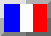Version francaise

## Measurement of neutrino mass

The mass of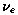, is measured since almost 50 years using mainly the beta decay. The continuous spectrum of beta decay electrons terminates at a maximal energy, which depends on the neutrino mass and on the emitting nucleus type. The measurement of this spectrum and the very difficult measurement of the nucleus recoil allows theoretically to determine the neutrino mass. The most precise measurements are made using the beta decay of tritium. From those measurements, we know today that theneutrino mass is less than 5.1 eV. Some recent measurements from 1995 and 1996 even let think that the neutrino mass squared is negative (that is neutrino mass is imaginary!). Some physicists even dare to do the hypothesis of a tachyonic neutrino (neutrino moving faster than light).

The mass of the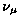neutrino can be obtained through the study of pion decay into muon. For now, this kind of measurement has given a mass less than 160 keV.

The mass of the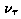neutrino is obtained through the study of tau decay or thanks to high energy experiments like Aleph at CERN which, through the study of decay channel of Z boson into tau allowed in 1995 to decrease at 24 MeV the superior limit on themass.

Back to main page

Last update: 26/06/1999 : http://wwwlapp.in2p3.fr/neutrinos/amassehe3.html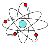Didier Verkindt